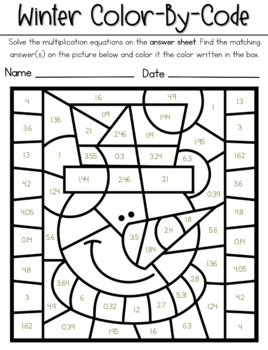5th Grade Winter Math Practice - Decimals5th
Subjects
Standards
Resource Type
Formats Included
• PDF
Pages
27 pages

Description

Help your 5th graders practice decimal operations with these real world, winter themed, no prep math pages! Students will practice estimating, comparing, adding, and subtracting decimals, as well as multiply and dividing decimals by whole numbers.

Money is a great way to practice decimal skills, and many of the problems in this set involve money! There are also differentiated pages to help students practice at a level that is appropriate for them.

This product includes:

• 2 pages of winter themed decimal word problems
• 2 differentiated pages for students to use decimal operations to shop a Winter Carnival Bake Sale
• 2 differentiated pages to help students plan a fundraiser
• 2 differentiated pages of problems to solve using information in a table
• 2 Color-By-Code pages: multiplying decimals by whole numbers and multiplying and dividing decimals by 10 and 100
• Answer keys for all pages

You might also be interested in these other 5th grade math resources:

Decimal Boom! Cards Bundle

5th Grade Problem of the Week

Valentine's Day Math Practice - Decimals

Don't forget to follow me to be notified when new resources are uploaded!

Total Pages
27 pages
Included
Teaching Duration
N/A
Report this Resource to TpT
Reported resources will be reviewed by our team. Report this resource to let us know if this resource violates TpT’s content guidelines.

Standards

to see state-specific standards (only available in the US).
Add, subtract, multiply, and divide decimals to hundredths, using concrete models or drawings and strategies based on place value, properties of operations, and/or the relationship between addition and subtraction; relate the strategy to a written method and explain the reasoning used.
Read, write, and compare decimals to thousandths.
Explain patterns in the number of zeros of the product when multiplying a number by powers of 10, and explain patterns in the placement of the decimal point when a decimal is multiplied or divided by a power of 10. Use whole-number exponents to denote powers of 10.